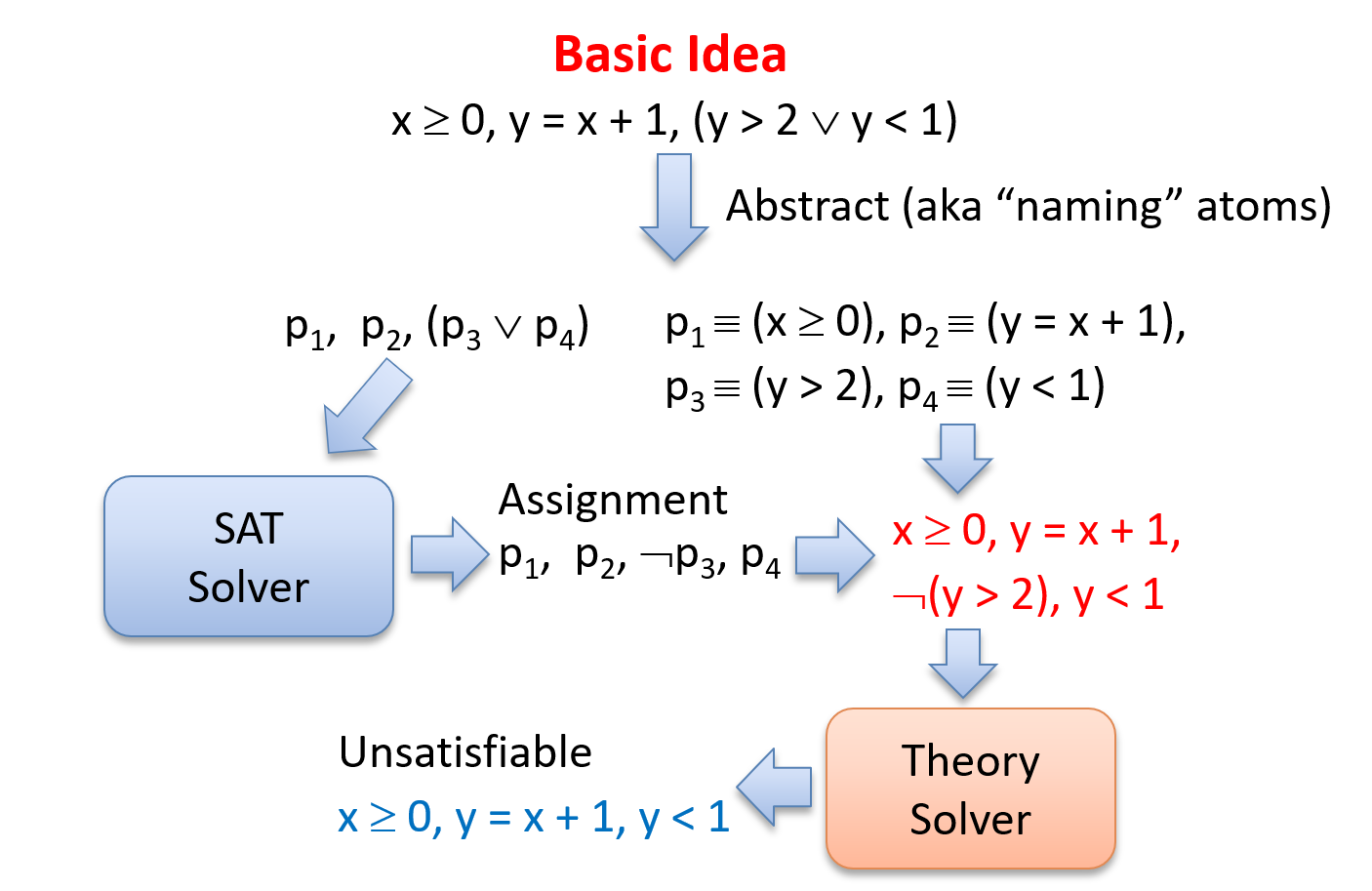## ATVA 2018

 Nikolaj Bjørner Microsoft Research nbjorner@microsoft.com http://theory.stanford.edu/~nikolaj/atva.html

## Ingredients of SMT Solving

 SAT SAT + Theories Theories Theories + Theories Programmability

SAT: Search Techniques

Theories: Solving Constraints over a theory

Theories + Theories: Integration of Theories

SAT + Theories: Integration of Theory Solvers with Search

Programmability: Programming Z3

### Z3 on GitHub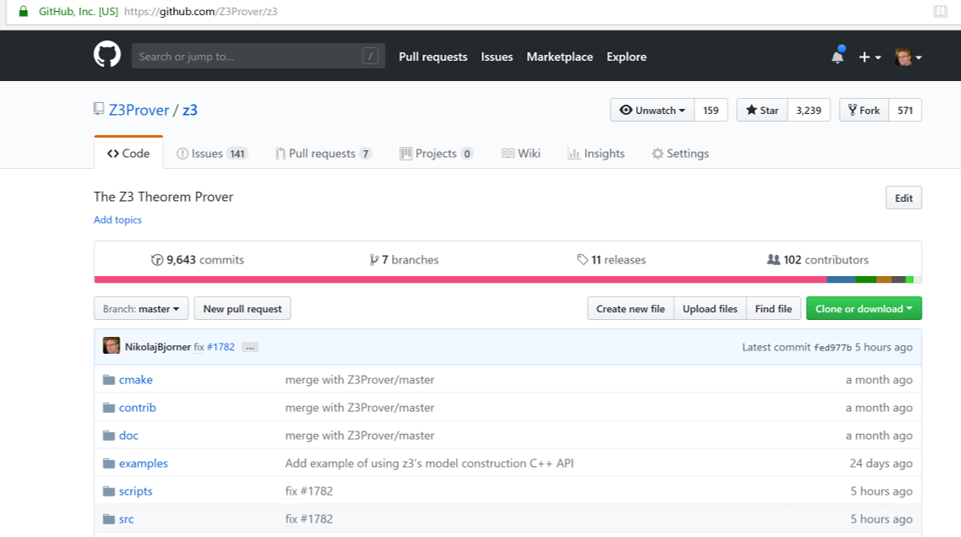### Recent developments in Z3

Current pre-release 4.8.0.0, July 3

A parallel cube & conquer mode

binding support

SAT solver with XOR, Cardinality and PB theories

SAT solver in-processing (ATE, ALA, ACCE, HBCA)

Model preservation across search resumption

SPACER/Horn engine by Arie Gurfinkel

New ILP engine by Lev Nachmanson

## Programming Z3

### SAT

  from z3 import *
Tie, Shirt = Bools('Tie Shirt')
s = Solver()
Or(Not(Tie), Shirt),
Or(Not(Tie), Not(Shirt)))
print(s.check())
print(s.model())

### SMT

  Z = IntSort()
f = Function('f', Z, Z)
x, y, z = Ints('x y z')
A = Array('A',Z,Z)
fml = Implies(x + 2 == y, f(Store(A, x, 3)[y - 2]) == f(y - x + 1))
solve(Not(fml))

### SMT-LIB and Python

 (set-logic QF_LIA)
(declare-const x Int)
(declare-const y Int)
(assert (> (+ (mod x 4) (* 3 (div y 2))) (- x y)))
(check-sat)

Python version:

 solve((x % 4) + 3 * (y / 2) > x - y)

### Python and SMT-LIB

It is also possible to extract an SMT-LIB2 representation of a solver state.

from z3 import *
x, y = Ints('x y')
s = Solver()
s.add((x % 4) + 3 * (y / 2) > x - y)
print(s.sexpr())

produces the output

(declare-fun y () Int)
(declare-fun x () Int)
(assert (> (+ (mod x 4) (* 3 (div y 2))) (- x y)))

### Accessing Expressions

x = Int('x')
y = Int('y')
n = x + y >= 3
print("num args: ", n.num_args())
print("children: ", n.children())
print("1st child:", n.arg(0))
print("2nd child:", n.arg(1))
print("operator: ", n.decl())
print("op name:  ", n.decl().name())
('num args: ', 2)
('children: ', [x + y, 3])
('1st child:', x + y)
('2nd child:', 3)
('operator: ', >=)
('op name:  ', '>=')

### Quantifiers

solve(y == x + 1, ForAll([y], Implies(y <= 0, x < y)))
• Is this satisfiable?

### Lambdas

m, m1 = Array('m', Z, Z), Array('m1', Z, Z)
def memset(lo,hi,y,m):
return Lambda([x], If(And(lo <= x, x <= hi), y, Select(m, x)))
solve([m1 == memset(1, 700, z, m), Select(m1, 6) != z])
Q = Array('Q', Z, B)
prove(Implies(ForAll(Q, Implies(Select(Q, x), Select(Q, y))), x == y))

## SMT Theories

### SAT + Theories

• Mainstream SMT solver architecture based on co-processor architecture to CDCL.

• Alternatives:

• Model Constructing SAT - instantiate values of non-Booleans
• MIP(T) - MIP solver is main engine
• Super-position

### CDCL(T) by example### CDCL(T) by example (2)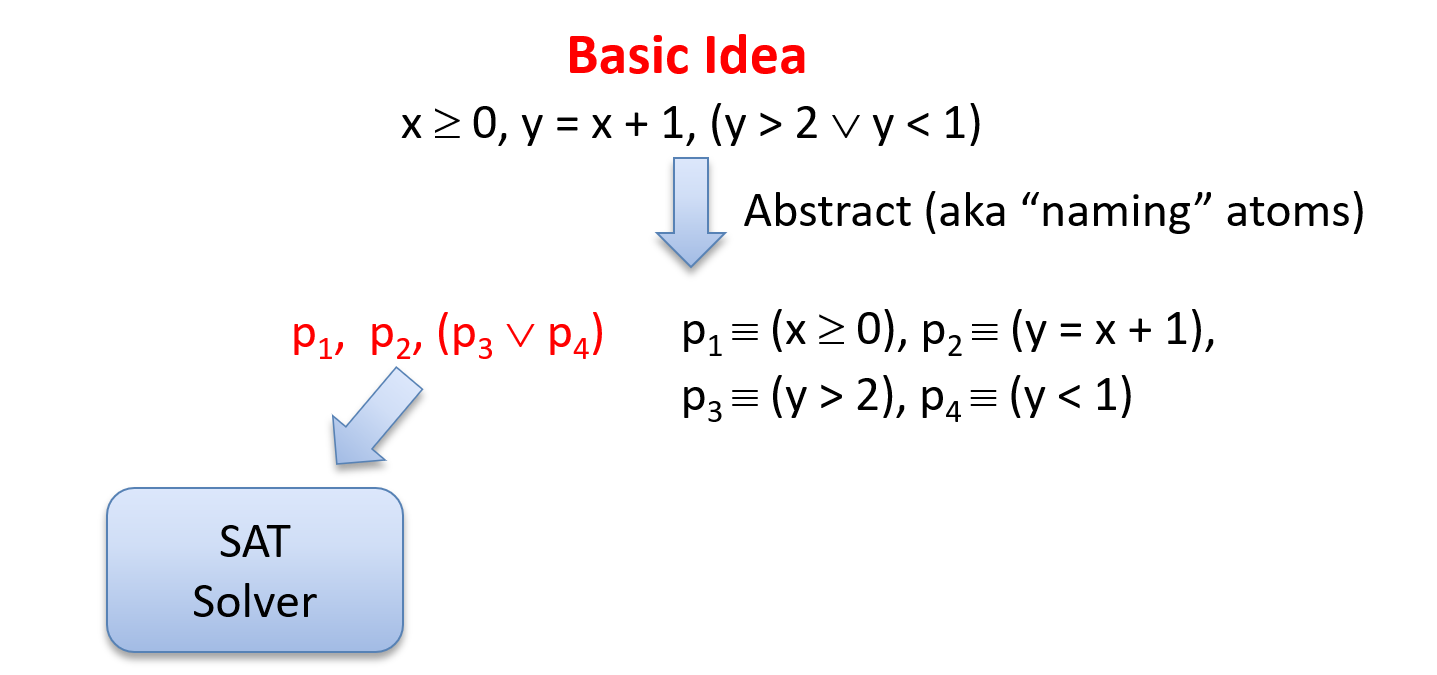### CDCL(T) by example (3)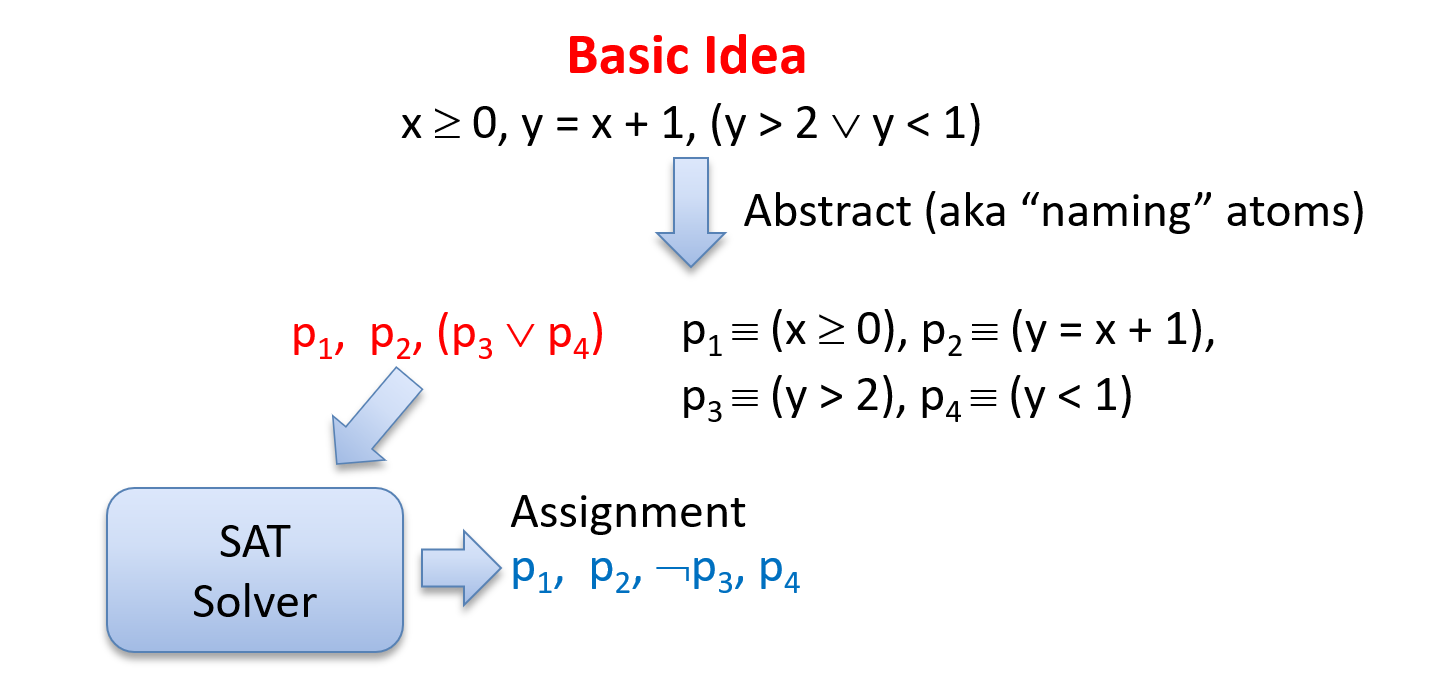### CDCL(T) by example (4)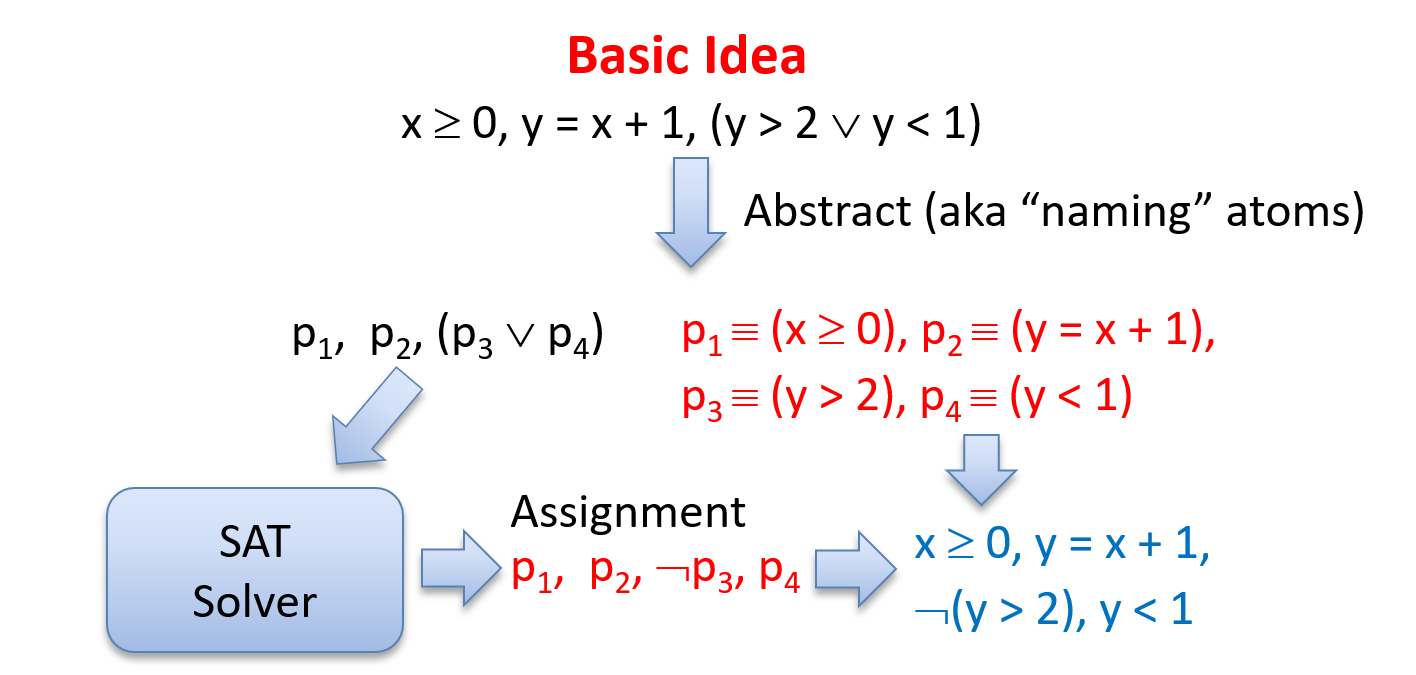### CDCL(T) by example (5)